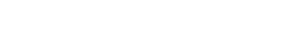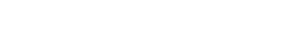3.2.4 Mole Calculations

Mole Calculations

The Mole

• The mole is one of the seven SI base units
• It is used to measure the amount of substance
• One mole is defined as follows:

The amount of substance containing exactly 6.02214076 × 1023 elementary entities (i.e. particles, atoms, molecules)

• At IB level, this number can be rounded to 6.02 × 1023
• One mole of gas contains a number of particles (atoms or molecules) equal to the Avogadro constant
• Therefore, to calculate the number of particles N in a gas, knowing the number of moles n, the following relationship must be used:

N = nNA

• Where:
• N = number of gas particles
• n = number of moles of gas (mol)
• NA = 6.02 × 1023 mol–1 (Avogadro constant)

Molar Mass

• The molar mass M of a substance is defined as the mass m of the substance divided by the amount (in moles) that substance
• The molar mass is calculated as follows:• Where:
• M = molar mass in g mol–1
• m = mass in grams (g)
• n = number of moles (mol)

Worked Example

Nitrogen is normally found in nature as a diatomic molecule, N2. The molar mass of nitrogen is 28.02 g mol–1.

Determine the mass of a nitrogen atom.

Step 1: Write down the relationship between the molar mass M and the massStep 2: Rearrange the equation to find the mass m of n = 1 mol of nitrogen

m = Mn

m = 28.02 g mol–1 × 1 mol

m = 28.02 g

Step 3: Identify the number of nitrogen atoms in 1 mol of nitrogen

• n = 1 mol of nitrogen contains a number of molecules equal to the Avogadro constant
• From the data booklet, NA = 6.02 × 1023

Nmolecules = nNA

Nmolecules = 6.02 × 1023

• Each nitrogen molecule contains 2 atoms of nitrogen
• n = 1 mol of nitrogen contains a number of atoms equal to twice the number of molecules

Natoms = 2 × Nmolecules = 2 × (6.02 × 1023)

Natoms = 1.2 × 1024

Step 4: Divide the mass of 1 mol of nitrogen m by the number of atoms in 1 mol of nitrogen Natoms in order to find the mass of a nitrogen atomMass of a nitrogen atom = 2.3 × 10–23 g

CloseClose